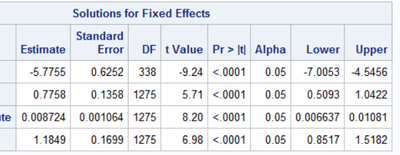## PROC GLIMMIX model both dichotomous and continuous independent variable

I am trying to model the data using a two-level model. Student nested in school. My dependent variable "pass" is dichotomously coded (1, 0). My  independent variable includes both dichotomously coded variable first and status (1, 0) and continuous variable "practice score"  as below

``````PROC GLIMMIX
DATA=pred METHOD=LAPLACE NOCLPRINT;
CLASS SCHOOLNUM;
MODEL PASS (EVENT="1")=first practice_score status/CL DIST=BINARY LINK=LOGIT SOLUTION;
RANDOM INTERCEPT/ SUBJECT=SCHOOLNUM S CL TYPE=VC;
COVTEST /WALD;
run;``````

Based on the fixed effects estimates as in the picture below, the probability of pass for first=1, status=1, is exp(-5.7755+0.7758+0.00872461.1849)/(1+exp(-5.7755+0.7758+0.008724+1.1849))=0.02175, which is too low from expected.Where was wrong with my SAS code above? Any help would be much appreciated. Thanks!

1 ACCEPTED SOLUTION

Accepted Solutions

## Re: PROC GLIMMIX model both dichotomous and continuous independent variable

Your formula is wrong. For each observation that you want a prediction, you want to use the values of the independent variables, which you are not doing. See Wikipedia or any textbook for the formula to predict: https://en.wikipedia.org/wiki/Multinomial_logistic_regression

The easiest way to predict the probability of passing is to use GLIMMIX, use the OUTPUT statement to have SAS compute the predicted probabilities. https://documentation.sas.com/doc/en/pgmsascdc/9.4_3.4/statug/statug_glimmix_syntax19.htm

--
Paige Miller
3 REPLIES 3

## Re: PROC GLIMMIX model both dichotomous and continuous independent variable

Your formula is wrong. For each observation that you want a prediction, you want to use the values of the independent variables, which you are not doing. See Wikipedia or any textbook for the formula to predict: https://en.wikipedia.org/wiki/Multinomial_logistic_regression

The easiest way to predict the probability of passing is to use GLIMMIX, use the OUTPUT statement to have SAS compute the predicted probabilities. https://documentation.sas.com/doc/en/pgmsascdc/9.4_3.4/statug/statug_glimmix_syntax19.htm

--
Paige Miller

## Re: PROC GLIMMIX model both dichotomous and continuous independent variable

@PaigeMiller  Thank you so much for your help!

## Re: PROC GLIMMIX model both dichotomous and continuous independent variable

The variable names and the values first=1 and status=1 make me wonder whether the FIRST and STATUS variables are binary classification variables? If so, you should add them to the CLASS statement.

Discussion stats
• 3 replies
• 185 views
• 1 like
• 3 in conversation